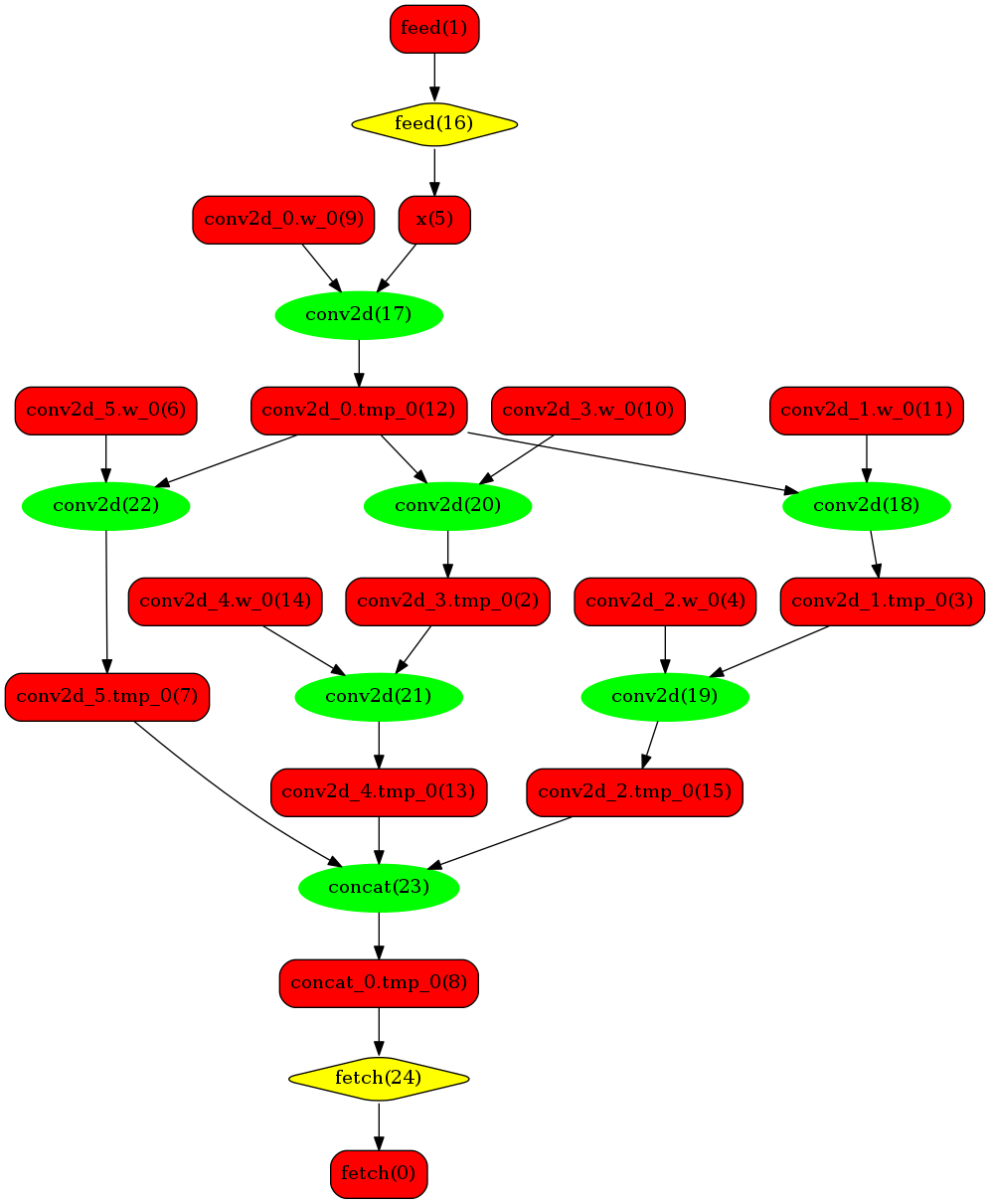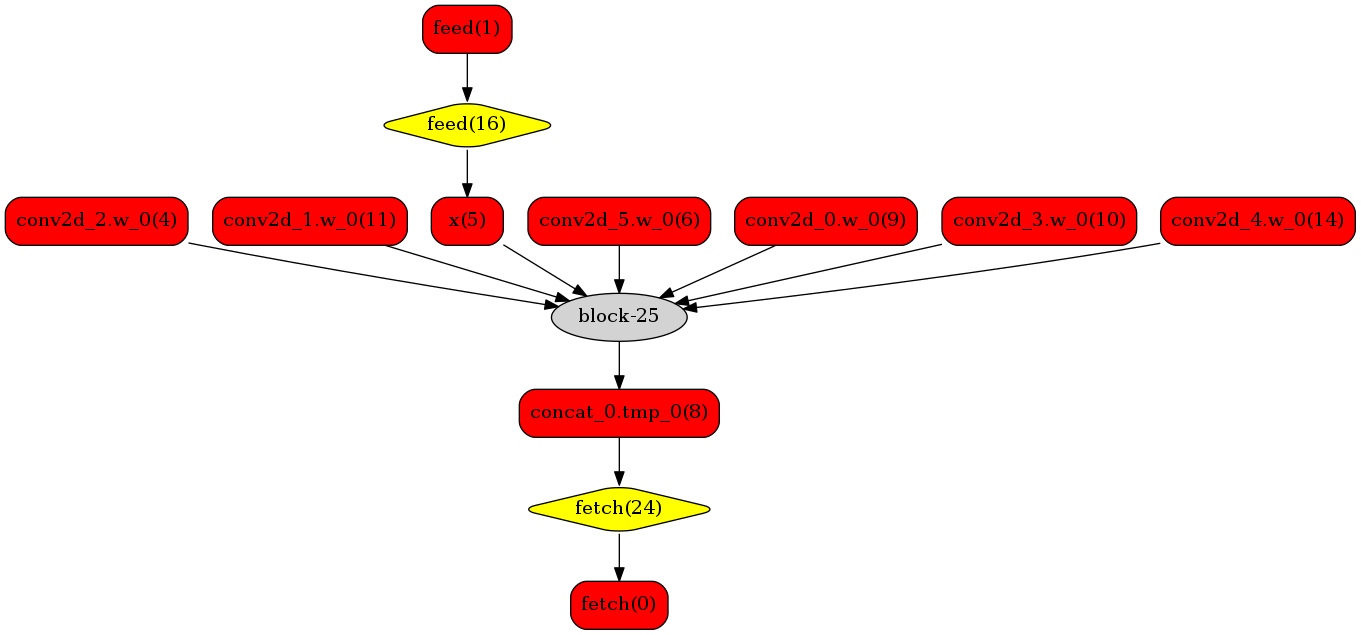## 概述¶

1）静态shape：

Mobilenetv1 yolov3 ICNET
Resnet50 SSD UNet
Resnext Faster-rcnn
Se-ResNext Retinanet
DPN

Fp16:

Calib Int8:

2）动态shape：

FCN Bert
Faster_RCNN Ernie

Fp16:

Calib Int8:

Note:

1. 从源码编译时，TensorRT预测库目前仅支持使用GPU编译，且需要设置编译选项TENSORRT_ROOT为TensorRT所在的路径。
2. Windows支持需要TensorRT 版本5.0以上。

## 一：环境准备¶

1）linux下通过pip安装

```# 该whl包依赖cuda10.1， cudnnv7.6， tensorrt6.0 的lib， 需自行下载安装并设置lib路径到LD_LIBRARY_PATH中
```

2）使用docker镜像

```# 拉取镜像，该镜像预装Paddle 1.8 Python环境，并包含c++的预编译库，lib存放在主目录～/ 下。

export CUDA_SO="\$(\ls /usr/lib64/libcuda* | xargs -I{} echo '-v {}:{}') \$(\ls /usr/lib64/libnvidia* | xargs -I{} echo '-v {}:{}')"
export DEVICES=\$(\ls /dev/nvidia* | xargs -I{} echo '--device {}:{}')
export NVIDIA_SMI="-v /usr/bin/nvidia-smi:/usr/bin/nvidia-smi"

```

3）手动编译 编译的方式请参照 编译文档

Note1： cmake 期间请设置 TENSORRT_ROOT （即TRT lib的路径）， WITH_PYTHON （是否产出python whl包， 设置为ON）选项。

Note2: 编译期间会出现TensorRT相关的错误。

```virtual ~IPluginFactory() {};
virtual ~IGpuAllocator() {};
```

```virtual ~IOptimizationProfile() noexcept = default;
```

## 二：API使用介绍¶

• 配置推理选项
• 创建predictor
• 准备模型输入
• 模型推理
• 获取模型输出

```import numpy as np

def create_predictor():
# config = AnalysisConfig("")
config = AnalysisConfig("./resnet50/model", "./resnet50/params")
config.switch_use_feed_fetch_ops(False)
config.enable_memory_optim()
config.enable_use_gpu(1000, 0)

# 打开TensorRT。此接口的详细介绍请见下文
config.enable_tensorrt_engine(workspace_size = 1<<30,
max_batch_size=1, min_subgraph_size=5,
precision_mode=AnalysisConfig.Precision.Float32,
use_static=False, use_calib_mode=False)

return predictor

def run(predictor, img):
# 准备输入
input_names = predictor.get_input_names()
for i,  name in enumerate(input_names):
input_tensor = predictor.get_input_tensor(name)
input_tensor.reshape(img[i].shape)
input_tensor.copy_from_cpu(img[i].copy())
# 预测
predictor.zero_copy_run()
results = []
# 获取输出
output_names = predictor.get_output_names()
for i, name in enumerate(output_names):
output_tensor = predictor.get_output_tensor(name)
output_data = output_tensor.copy_to_cpu()
results.append(output_data)
return results

if __name__ == '__main__':
pred = create_predictor()
img = np.ones((1, 3, 224, 224)).astype(np.float32)
result = run(pred, [img])
print ("class index: ", np.argmax(result))
```

```config.enable_tensorrt_engine(
workspace_size = 1<<30,
max_batch_size=1, min_subgraph_size=5,
precision_mode=AnalysisConfig.Precision.Float32,
use_static=False, use_calib_mode=False)
```

• workspace_size，类型：int，默认值为1 << 30 （1G）。指定TensorRT使用的工作空间大小，TensorRT会在该大小限制下筛选最优的kernel执行预测运算。
• max_batch_size，类型：int，默认值为1。需要提前设置最大的batch大小，运行时batch大小不得超过此限定值。
• precision_mode，类型：AnalysisConfig.Precision, 默认值为 AnalysisConfig.Precision.Float32。指定使用TRT的精度，支持FP32（Float32），FP16（Half），Int8（Int8）。若需要使用Paddle-TRT int8离线量化校准，需设定precision为 AnalysisConfig.Precision.Int8 , 且设置 use_calib_mode 为True。
• use_static，类型：bool, 默认值为False。如果指定为True，在初次运行程序的时候会将TRT的优化信息进行序列化到磁盘上，下次运行时直接加载优化的序列化信息而不需要重新生成。

### Int8量化预测¶

1. 产出量化模型

1. 使用TensorRT自带Int8离线量化校准功能。校准即基于训练好的FP32模型和少量校准数据（如500～1000张图片）生成校准表（Calibration table），预测时，加载FP32模型和此校准表即可使用Int8精度预测。生成校准表的方法如下：
• 指定TensorRT配置时，将 precision_mode 设置为 AnalysisConfig.Precision.Int8 并且设置 use_calib_modeTrue

```config.enable_tensorrt_engine(
workspace_size=1<<30,
max_batch_size=1, min_subgraph_size=5,
precision_mode=AnalysisConfig.Precision.Int8,
use_static=False, use_calib_mode=True)
```

2. 加载量化模型进行Int8预测

```config.enable_tensorrt_engine(
workspace_size=1<<30,
max_batch_size=1, min_subgraph_size=5,
precision_mode=AnalysisConfig.Precision.Int8,
use_static=False, use_calib_mode=True)
```

```config.enable_tensorrt_engine(
workspace_size=1<<30,
max_batch_size=1, min_subgraph_size=5,
precision_mode=AnalysisConfig.Precision.Int8,
use_static=False, use_calib_mode=False)
```

### 运行Dynamic shape¶

```config.enable_tensorrt_engine(
workspace_size = 1<<30,
max_batch_size=1, min_subgraph_size=5,
precision_mode=AnalysisConfig.Precision.Float32,
use_static=False, use_calib_mode=False)

min_input_shape = {"image":[1,3, 10, 10]}
max_input_shape = {"image":[1,3, 224, 224]}
opt_input_shape = {"image":[1,3, 100, 100]}

config.set_trt_dynamic_shape_info(min_input_shape, max_input_shape, opt_input_shape)
```

## 三：测试样例¶

• Python 样例请访问此处 链接
• C++ 样例地址请访问此处 链接